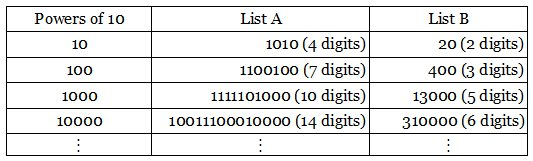# Two Lists

Write out the positive powers of 10 in both base 2 and base 5:Now for any integer n > 1, we’ll find exactly one number of length n somewhere on the two lists. They contain one 3-digit number, one 4-digit number, and so on forever — if n = 100 we find a 100-digit number in the 30th position on the base 2 list.

(This result first appeared in the 1994 Asian Pacific Mathematics Olympiad. I found it in Ravi Vakil’s A Mathematical Mosaic.)

Two further curious lists: If we write out the triangular numbers, those in positions 3, 33, etc. show a pattern:

T(3) = 6
T(33) = 561
T(333) = 55611
T(3,333) = 5556111
T(33,333) = 555561111
T(333,333) = 55555611111

Similarly:

T(6) = 21
T(66) = 2211
T(666) = 222111
T(6,666) = 22221111
T(66,666) = 2222211111
T(666,666) = 222222111111

(Thanks, Larry.)